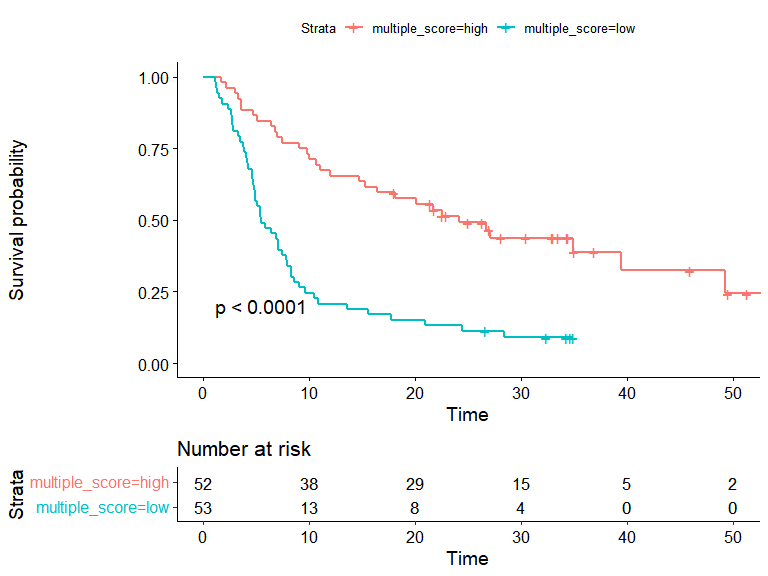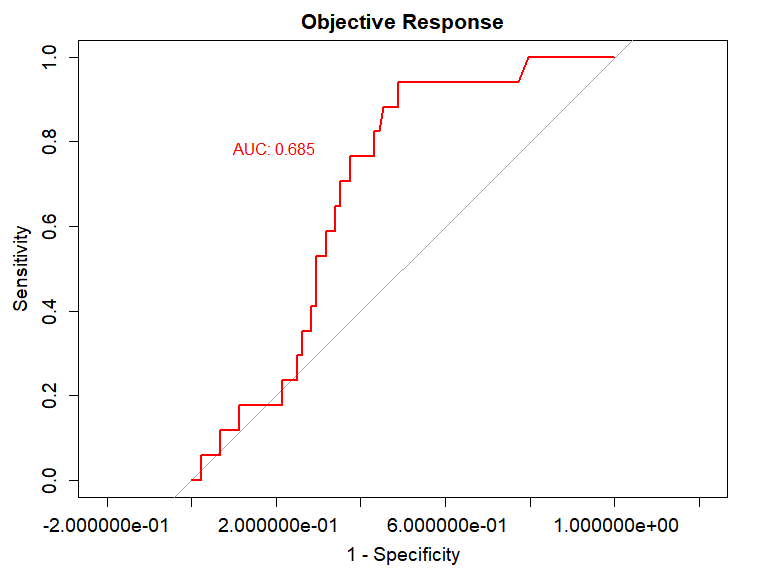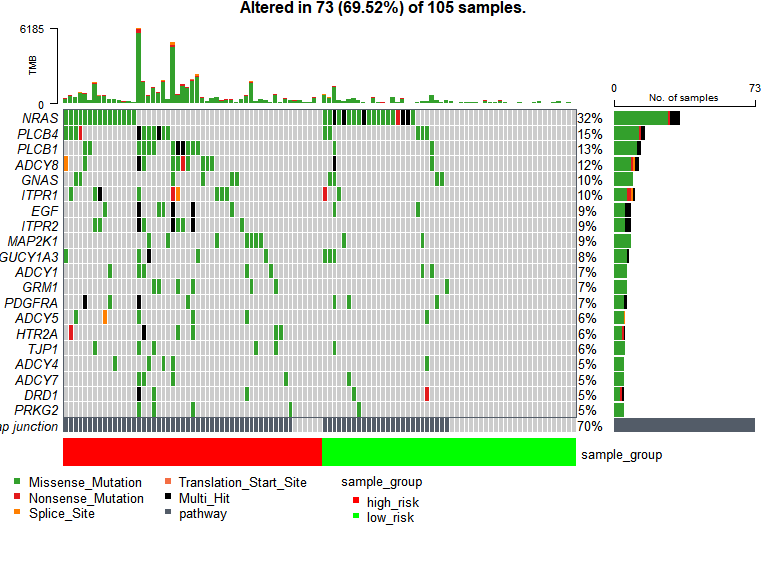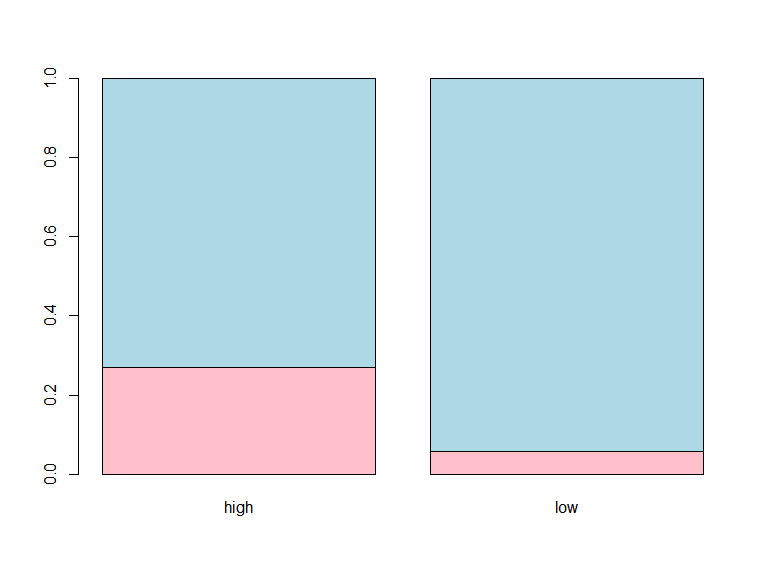# PMAPscore

#### 2022-04-12

library(PMAPscore)
#>
#> 

# Introduction

Somatic mutations play an important role in cancer development and progression, and mutational profiling is used far more commonly than other ‘omics’ analyses in clinical practice.And nowadays immunotherapy has become a powerful clinical strategy for treating cancer.Tumor mutation burden (TMB) is a potential biomarker in immunotherapy.However, it is still insufficient to only rely on TMB to predict the response of immunotherapy.This package attempts to develop a new pathway-based mutation accumulate perturbation score (PMAPscore) which can be used as an auxiliary factor of TMB to further improve the accuracy of immunotherapy response prediction,efficiently using somatic mutations files in from either cBioProtal sources or any in-house studies as long as the data is in MAF format. Besides, we develop a multiple machine learning method using sample PMAPscore profiles to identify dysregulated cancer-specific dysregulated signal pathways, which can be a biomarker of prognostic and predictive for cancer immunotherapy.

# MAF field requirements

MAF files contain many fields ranging from chromosome names to cosmic annotations. However, most of the analysis in PMAPscore uses the following fields.

• Mandatory fields:
Hugo_Symbol,
Entrez_Gene_Id,
Variant_Classification,
Tumor_Sample_Barcode.

Complete specification of MAF files can be found on NCI GDC documentation page.

# Installation

install.packages("PMAPscore")
library(PMAPscore)

# Overview of the package

The PMAPscore package is a Bioinformatics tool to identify cancer-specific dysregulated signal pathway. And PMAPscore functions can be categorized into mainly Visualization and Analysis modules. Each of these functions and a short description is summarized as shown below:

1.Get the mutation status of all genes in each sample.
2.Calculate the mutation cumulative score of each pathway PMAPscore.
3.Identify cancer-specific dysregulated signal pathways. 4.Calculate the risk score of each sample and classify the samples according to the threshold. 5.Visualization results:
5.1 Plot patients’ Kaplan-Meier Survival Curves.
5.2 Plot the ROC curve. 5.3 Plot patient-specific dysfunction pathways’ waterfall plots. 5.4 Plot the histogram of the patients’ drug response.

## Get the mutation status of all genes in each sample

We downloaded patients’ mutation data from the cBioPortal database in MAF format. About the mutation status of a specific gene in a specific sample, we converted MAF format data into a mutation status matrix, in which every row represents the gene and every column represents the sample. In our study, we only extract the nonsilent somatic mutations (nonsense mutation, missense mutation, frame-shift indels, splice site, nonstop mutation, translation start site, inframe indels) in protein-coding regions.The function get_mut_status in the PMAPscore package can implement the above process. Take simulated melanoma data as an example, the command lines are as follows:

#load the mutation annotation file
data("maf_data")
#perform the function 'get_mut_status'
mut_status<-get_mut_status(maf_data=maf_data,nonsynonymous = TRUE)
#view the first five lines of mut_status matrix
mut_status[1:5,1:5]
#>          Pat02 Pat03 Pat04 Pat06 Pat07
#> MIB2         1     0     0     0     0
#> NASP         1     0     0     0     0
#> C1orf173     1     0     0     0     0
#> SLC44A5      1     0     0     0     0
#> OR10R2       1     0     0     0     0

## Calculate the perturbation score of each pathway PMAPscore.

According to the mutation status in each sample and the position of gene in the signal pathway,we obtain the PMAPscore of each pathway in a single sample by accumulating an additive sum of gene/protein relationship coefficients.The function get_pfs_score in the PMAPscore package can implement the above process. The calculation formula is as follows:
###gene perturbation’s score: $GMPscore(g_{i})=△E(g_{i})+\sum_{i=1}^N β_{ij}\frac{GMPscore(g_{j})}{N_{ds(g_{j})}}\tag{1}$
where $$△E(g_{i})$$ is the $$i$$ th gene’s mutation status(1 is mutant and 0 is non-mutant); $$β_{ij}$$ is the relationship between genes gi and gj,if the gene j is directly interacted with the gene i according to the pathway description, $$β_{ij} = 1$$, else is 0; $$N_{ds(g_{j})}$$ is the number of genes downstream of the gene $$j$$.The $$GMPscore(g_{i})$$ denotes the gene $$i$$ mutation perturbation score.The $$GMPscore(g_{j})$$ denotes the mutation perturbation score of upstream gene $$j$$ of gene $$i$$.

###pathway mutation perturbation’s score: $PMAPscore(p_{i})=\frac{\sum_{k=1}^NGMPscore(g_{k})}{N_{de}}$ where$$N$$ is the total number of genes in pathway $$i$$;$$GMPscore(g_{k})$$ indicates mutation the perturbation score of genes in the pathway $$i$$;$$N_{de}$$ denotes the number of genes that have mutated in the pathway $$i$$;$$PMAPscore(p_{i})$$ indicates the perturbation score of pathway $$i$$.

#Method of obtaining data
data(mut_status,gene_Ucox_res,gene_symbol_Entrez)
#calculate the pfs_score of single sample
pfs_score<-get_pfs_score(mut_status[,1:2],percent=0.03,gene_Ucox_res,gene_symbol_Entrez)
#view the first five lines of pfs_score matrix
pfs_score[1:5,1:2]

## Identify dysregulated cancer-specific signal pathways.

In order to identify dysregulated cancer-specific signal pathways, we develop a multiple machine learning method using pfs_score. The multiple machine learning method consists of three sequential steps, as follows:

1. Wilcoxon test was used to tentatively identify differentially PMAPscore pathways between death and alive sample groups.
2. Univariate Cox regression was used to further select the prognosis-related features.
3. Finally,Lasso regression model was also performed to select the potential predictivel features.

The function get_final_signature in the PMAPscore package can implement the above process.

#load pfs_score and survival data
data(pfs_score,sur)
# filter the survival-related pathways
final_signature<-get_final_signature(pfs_score,sur)
#view the final_character
final_signature
#>  "Gap junction"
#>  "JAK-STAT signaling pathway"
#>  "EGFR tyrosine kinase inhibitor resistance"
#>  "Fanconi anemia pathway"
#>  "Cytokine-cytokine receptor interaction"
#>  "HIF-1 signaling pathway"
#>  "Taste transduction"

##Calculate the risk score of each sample and classify the samples according to the threshold. The function get_sample_classification can get the risk score of a sample according to the mutation status of the genes contained in the sample, and classify the sample by the threshold we set.

#Load sample mutation data
data(mut_sam,gene_Ucox,symbol_Entrez,path_cox_data,sur,path_Ucox_mul,sig)
#Perform the function get_sample_classification
get_sam_cla(mut_sam,gene_Ucox,symbol_Entrez,path_cox_data,sur,path_Ucox_mul,sig,cut_off=-0.986)
#>       sample  risk_score          class
#> Pat02 "Pat02" "-1.66298046373325" "low"
#> Pat03 "Pat03" "0"                 "high"
#class_res

## Visualization results

1. The function ‘get_km_survival_curve’ is used to draw Kaplan-Meier survival curves based on PMP-related risk score. The risk score is generated by the signature’s PMPAscore and the coefficient of multivariate cox regression. The command lines are as follows:

#Load the data
data(km_data)
#Drawing Kaplan-Meier Survival Curves.
get_km_survival_curve(km_data,cut_point,TRAIN = TRUE,risk.table=TRUE)#> 0.9243611
1. The function ‘get_roc_curve’ is used to plot a ROC curve.

#Get the data of ROC curve
data(roc_data)
#Drawing ROC Curves
get_roc_curve(roc_data,print.auc=TRUE,main="Objective Response")
#> Setting levels: control = 0, case = 1
#> Setting direction: controls < cases(3) The function ‘get_Oncoplots’ takes output generated by read.maf and draws a GenePathwayOncoplots

#obtain the risksciore
data(km_data)
risk_score<-km_data\$multiple_score
names(risk_score)<-rownames(km_data)
cut_off<-median(risk_score)
data(final_signature,path_gene,mut_status,maffile)
#draw an GenePathwayOncoplots
get_Oncoplots(maffile,path_gene,mut_status,risk_score,cut_off,final_signature,"Gap junction")
#> Gap junction not found in MAF. Excluding it..#> --Possible FLAGS among top ten genes:
#>   TTN
#>   MUC16
#>   USH2A
#> -Processing clinical data
1. The function ‘get_response_plot’ is used to draw a histogram of the patient’s drug response.The command lines are as follows:

#Load the data
data(km_data,response)
#Drawing the histogram.
get_response_plot(km_data,response,cut_point,TRAIN=TRUE)#>
#>  Pearson's Chi-squared test with Yates' continuity correction
#>
#> data:  res_matrix1
#> X-squared = 7.2485, df = 1, p-value = 0.007096